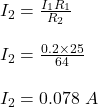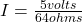## Two resistors, R1 = 25.0 ohms and R2 = 64.0 ohms, are connected in parallel. R1 has a 0.200 A current fl

Question

Two resistors, R1 = 25.0 ohms and

R2 = 64.0 ohms, are connected in

parallel. R1 has a 0.200 A current

flowing through it. What is the current

through R2?

Domomhor resistors in norollal

in progress 0
3 months 2021-07-25T17:50:18+00:00 2 Answers 4 views 0

the current through R₂ is 0.078 A

Explanation:

Given;

resistance of the first resistor, r₁ = 25 ohms

resistance of the second resistor, r₂ = 64 ohms

current through the first resistor, I₁ = 0.2 A

The voltage passing through each resistor is constant since they are connected parallel to each other.

V = I₁R₁ = I₂R₂Therefore, the current through R₂ is 0.078 A

The current through R2 is 0.078125 amperes.

Explanation:

Ohm’s law is the relationship between electrical conductors and their resistance, which establishes that the current that passes through the conductors is proportional to the voltage applied to them.

Ohm’s Law relates the magnitudes of voltage, resistance, and intensity as follows:

V=I*R

where V is the electric potential in volts, I is the current in amperes, and R is the resistance in ohms.

R1 has a 0.200 amperes current  flowing through it and a resistante of 25 ohms.

So:

V=0.200 amperes* 25 ohms

Solving:

V=5 volts

Now, for resistance R2 you know the value of the voltage (5 volts) and the value of its resistance R2 = 64 ohms. So:

5 volts= I* 64 ohms

Solving:I= 0.078125 amperes

The current through R2 is 0.078125 amperes.